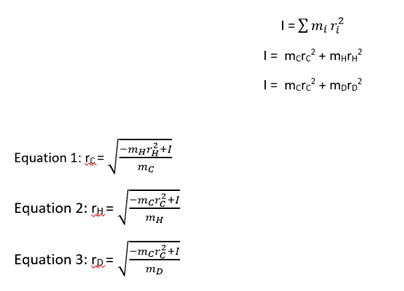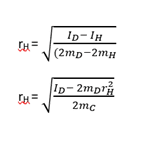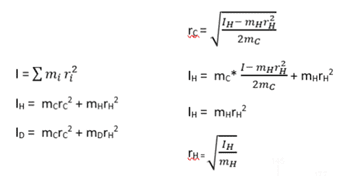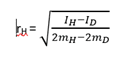# Need Help with Bond Length Calculations of Acetylene

• Chemistry
Popz
Homework Statement:
I have done most part of the data analysis. I am stuck with rearranging the equation in the correct way.
Relevant Equations:
see below
Hey!

I am trying to determine the bond length of acetylene by analysing the vibrational spectrum of both H-Acetylene and D-Acetylene. I was able to calculate both the rotational constant and the moment of inertia but am stuck now as to how I get to the correct solution.On the left is the original moment of inertia equation for polyatomic molecules which I have expanded to be specific for acetylene.
Then, on the right side are my rearrangements for the bond length of the respective molecules. I have been told that this is solvable by using two simultaneous equations - the moment of inertia for H and D. But I don't know how to solve this in practice.However, I have been told that this is (probably) the correct equation. But I don't know how I get to this point.
I understand that the basic rearrangement equals r = √(I/2m) but, for example, I don't know why r(D) - r(H) would equal r(H) if that's what the first equation implies?​

Hope that's not too confusing.
Thanks!

Homework Helper
You are over-complicating things with your square-root equations. In the equations for the moments of inertia (label them IH and ID so you don't start thinking they're the same) you have two simultaneous linear equations in the unknowns rC2 and rH2 (assuming rD = rH). Solve for these and then root them. Oh, and the equations are missing a factor of 2.

Popz
Thank you! I had an attempt following what you said. It would be great if you could double-check if I'm on the right track as it has truly been a while since I last have done this kind of maths.So I labeled the equations accordingly and then subsituted rc in the H-Inertia equation which gave me a simpler equation for rh. If this is correct, how do I move forward? Do I substitute (I/m) for rh in the rc equation on the top right?

Also out of curiosity, is it always the case that the isotope of a molecule doesn't affect bond length?

Homework Helper
I have no idea what rubbish you're doing in the right hand column.

You have two equations (which you are still writing wrongly):
IH = 2mCrC2 + 2mHrH2
ID = 2mCrC2 + 2mDrH2
You can do a simple subtraction to get
ID - IH = 2(mD - mH)rH2
and solve for rH, and substitute back in the equation for rC.
Note that the question asks for the bond lengths, not rH and rC.

Popz
Thanks!
From that on I calculated thenWhich is the same as the equation I posted in the original post except that the values for D and H are swapped. Is this correct or have I overlooked something?

For rc I then come to the same conclusion as the equation in the original post too.